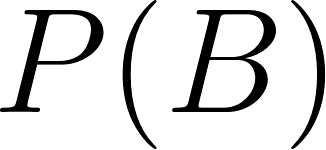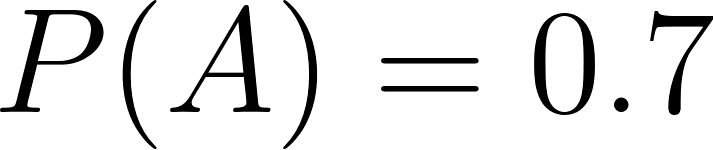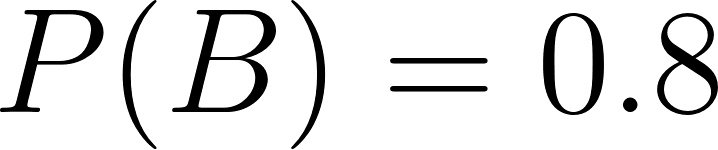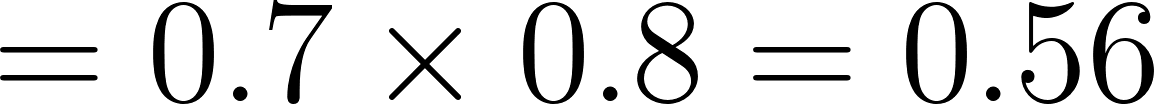×
Get Full Access to Precalculus With Trigonometry: Concepts And Applications - 1 Edition - Chapter 9-10 - Problem 19
Get Full Access to Precalculus With Trigonometry: Concepts And Applications - 1 Edition - Chapter 9-10 - Problem 19

×

# Indy 500 Problem: Hezzy Tate has a 70% probability of finishing in the top ten places inISBN: 9781559533911 468

## Solution for problem 19 Chapter 9-10

Precalculus with Trigonometry: Concepts and Applications | 1st Edition

• Textbook Solutions
• 2901 Step-by-step solutions solved by professors and subject experts
• Get 24/7 help from StudySoup virtual teaching assistantsPrecalculus with Trigonometry: Concepts and Applications | 1st Edition

4 5 1 361 Reviews
27
4
Problem 19

Indy 500 Problem: Hezzy Tate has a 70% probability of finishing in the top ten places in the Indianapolis 500-car race. His wife, Aggie, has an 80% probability of finishing in the top ten. a. What is the probability that Hezzy and Aggie both finish in the top ten? b. The probability that Hezzy finishes in the top ten but Aggie does not is 0.14. Show how you could calculate this number. c. What is the probability that Aggie finishes in the top ten but Hezzy does not? d. What is the probability that neither of them finishes in the top ten? e. The Tates decide to go on a vacation to spend their winnings. They will spend the following amounts, depending on which one or ones win. H and A: Hawaii, $8000 H, not A: California,$3000 A, not H: Florida, $4000 Neither: Stay home,$0 Calculate the mathematically expected

Step-by-Step Solution:

Problem 19

Indy 500 Problem: Hezzy Tate has a 70% probability of finishing in the top ten places in the Indianapolis 500-car race. His wife, Aggie, has an 80% probability of finishing in the top ten. a. What is the probability that Hezzy and Aggie both finish in the top ten? b. The probability that Hezzy finishes in the top ten but Aggie does not is 0.14. Show how you could calculate this number. c. What is the probability that Aggie finishes in the top ten but Hezzy does not? d. What is the probability that neither of them finishes in the top ten? e. The States decide to go on a vacation to spend their winnings. They will spend the following amounts, depending on which one or ones win. H and A: Hawaii, $8000 H, not A: California,$3000 A, notcH: Florida, $4000 Neither: Stay home,$0 Calculate the mathematically expected.

Step-by-step solution

Step 1 of 5

a)

Letis the probability that Hezzy Tate has finished in the top ten places in the Indianapolis 500-car race.is the probability that Aggie has finished in the top ten places in the Indianapolis 500-car race.Ifandare independent use the ruleThe probability that Hezzy and Aggie both finish in top tenStep 2 of 5

Step 3 of 5

##### ISBN: 9781559533911

This textbook survival guide was created for the textbook: Precalculus with Trigonometry: Concepts and Applications, edition: 1. The full step-by-step solution to problem: 19 from chapter: 9-10 was answered by , our top Calculus solution expert on 03/16/18, 04:16PM. Since the solution to 19 from 9-10 chapter was answered, more than 225 students have viewed the full step-by-step answer. Precalculus with Trigonometry: Concepts and Applications was written by and is associated to the ISBN: 9781559533911. The answer to “Indy 500 Problem: Hezzy Tate has a 70% probability of finishing in the top ten places in the Indianapolis 500-car race. His wife, Aggie, has an 80% probability of finishing in the top ten. a. What is the probability that Hezzy and Aggie both finish in the top ten? b. The probability that Hezzy finishes in the top ten but Aggie does not is 0.14. Show how you could calculate this number. c. What is the probability that Aggie finishes in the top ten but Hezzy does not? d. What is the probability that neither of them finishes in the top ten? e. The Tates decide to go on a vacation to spend their winnings. They will spend the following amounts, depending on which one or ones win. H and A: Hawaii, $8000 H, not A: California,$3000 A, not H: Florida, $4000 Neither: Stay home,$0 Calculate the mathematically expected” is broken down into a number of easy to follow steps, and 151 words. This full solution covers the following key subjects: . This expansive textbook survival guide covers 106 chapters, and 2321 solutions.

Unlock Textbook Solution